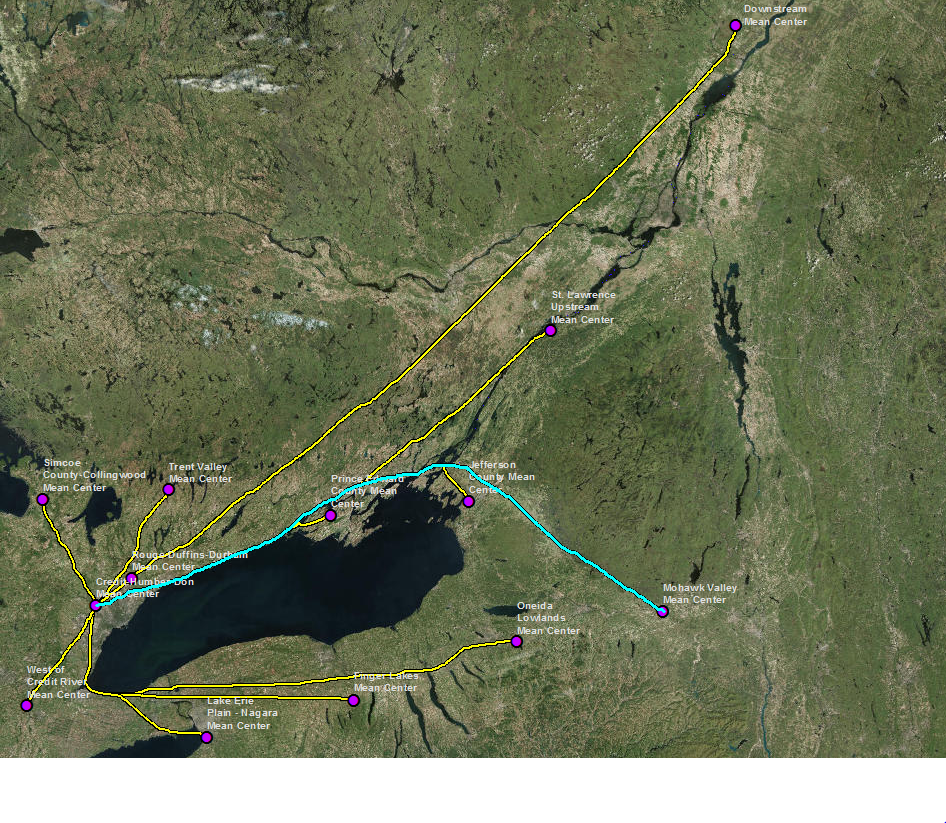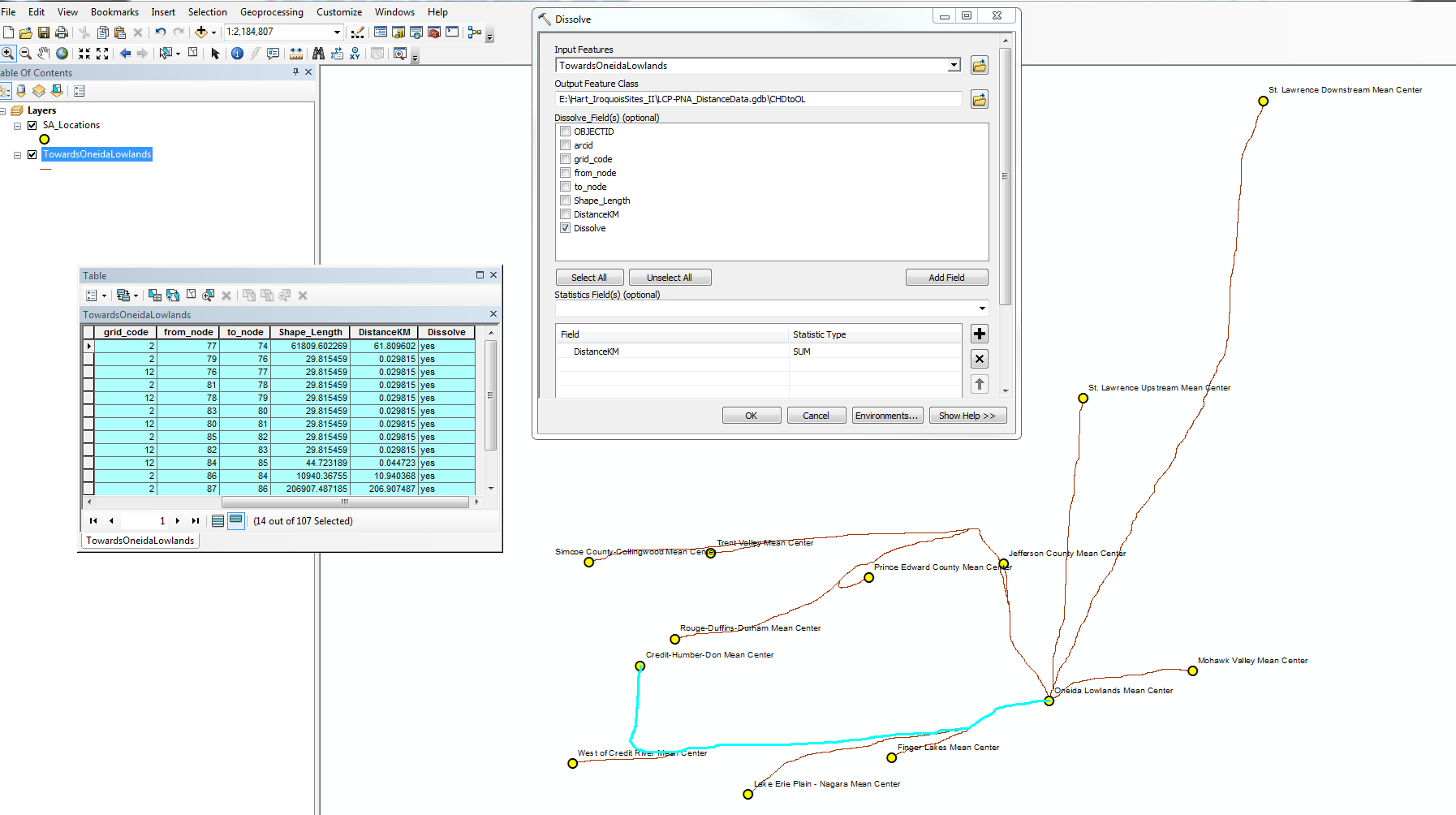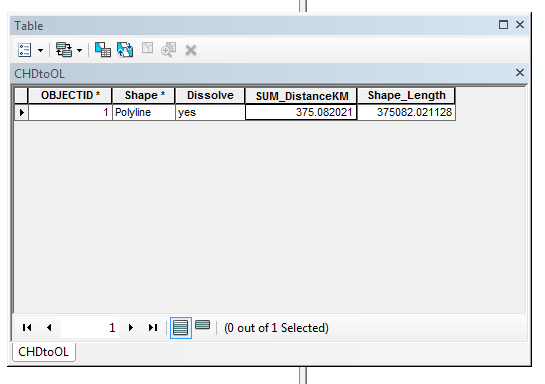# Calculating distance between points along (segmented) least cost path

1142
5
06-12-2018 02:57 PM
HighlightedABOVE SCREEN SHOT -- I have two datasets: a point feature class representing destinations, and a polyline feature class representing the fastest route to one singular destination from every other point. What I would like to do now is automatically calculate the total distance of each path from a single origin point to the destination point. I can do this manually by selectingn all the segments that comprise a path as shown above, and summarizing the shape_length field in the attribute table, but it seems like this should be a fairly straightforward task for ArcGIS.

I've read other Geonet messages asking similar questions, and have successfully converted my polyline to a route [using Create Routes tool in the Linear Referencing Toolbox] and then attempted to use the Locate Features Along a Route tool, but the resulting table did not contain measurements that made any sense (note: the polyline/route feature class actually contains 188 line segments rather 12 as what appears to be illustrated above)

Any guidance/suggestions would be greatly appreciated!

Susan

5 Replies
Highlighted
byMVP Esteemed Contributor

Is everything in a projected coordinate system?

What does the attribute table look like?

I am wondering if you want to Dissolve the bits/pieces of one route into one shape to save you having to Summarize the tabular results.

Results in planar units can also be derived from the Add XY geometry tools

Highlighted

Hi Dan,

Thanks very much for the reply (and my apologies for the delay!) Here are the answers to your queries:

a) everything is in a projected coordinate system.

b) screen shot of attribute table below:c) I have been manually selecting segments and using the DISSOLVE tool now rather than statistics and producing an individual line; this actually requires additional steps and time than using Statistics (but I've since been asked to determine the highest and lowest elevation along each of these, so I need the output polylines this tool creates anyway).

Here's a restatement of the issue:

ArcGIS has a tool - POINT DISTANCE - to generate a table very quickly of distances between point features, but it only calculates straight line distance. I have used this numerous times to produce straight line distance matrixes --  export out the table and import into Excel, and create a Pivot Table.

Using RASTER CALCULATOR, COST DISTANCE and the COST PATH tools (and converting the resulting Least Cost Path raster to polyline feature) do generate distances and place them in an attribute table associated with the least cost paths, but even the simplest paths (for example, one LCP dataset called "Walking Towards St. Lawrence Downstream" – similar to what is shown above, but with reasonably straight lines from each origin to the destination) are highly segmented, because the 13 rasters I created produced 12 lines moving from an origin to a destination; several of these 12 lines overlap.  Distance is calculated for each segment, but not the total distance between the points. In this example,  instead of 12 lines (what it appears to be), there are actually 123 different polyline segments. (If I had chosen to calculate one line at a time – between one origin point and one destination – and presumably producing a single, unsegmented line, the number of rasters would have exponentially increased. Creating one raster takes hours).

So, what I was doing before (to produce the distance matrix I need for this study)  was selecting all the segments that comprise a single least cost path, and using a  “calculate statistics” (a fairly automated process) and copying and pasting the sum in Excel – this is somewhat time-consuming, definitely tedious, but it’s pretty straightforward, and far, far less time-consuming than if I had done one line at a time as noted above. I'm still doing the same thing, essentially - manual selection of all the segments I need, but now producing a new feature polyline with the selected segments and the DISSOLVE tool, and deriving the distance I need (between a single origin point and a single destination)  by using the statistics field option (SUM) in the DISSOLVE tool (screen shot below of the tool and the attribute table of the resulting polyline):So, basically, I'm looking for something comparable to POINT DISTANCE, but to calculate the distance between two points along a least cost path.

Thank you again.

Susan

Highlighted

Did you find a solve for your question? is there a way to do this?

Highlighted

Hi David,

I did not get an answer to the original question about automating the process -- my "solution" was to do what I described in the reply to Dan Patterson dated June 25, 2018:

I have been manually selecting segments and using the DISSOLVE tool now rather than statistics and producing an individual line; this actually requires additional steps and time than using Statistics (but I've since been asked to determine the highest and lowest elevation along each of these, so I need the output polylines this tool creates anyway).

Susan

Highlighted

Thank you Susan for your assistance with this problem. Your description was very useful for me.